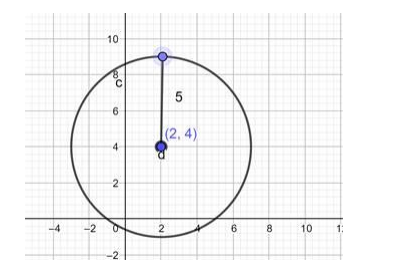# Find the equation of a circle with`
Question:

Find the equation of a circle with Centre $(2,4)$ and radius 5

Solution:

The general form of the equation of a circle is:

$(x-h)^{2}+(y-k)^{2}=r^{2}$

Where, (h, k) is the centre of the circle.

r is the radius of the circle.

Substituting the centre and radius of the circle in he general form:

$\Rightarrow(x-2)^{2}+(y-4)^{2}=5^{2}$

$\Rightarrow(x-2)^{2}+(y-4)^{2}=25$Ans; equation of a circle with Centre (2, 4) and radius 5 is:

$\Rightarrow(x-2)^{2}+(y-4)^{2}=25$